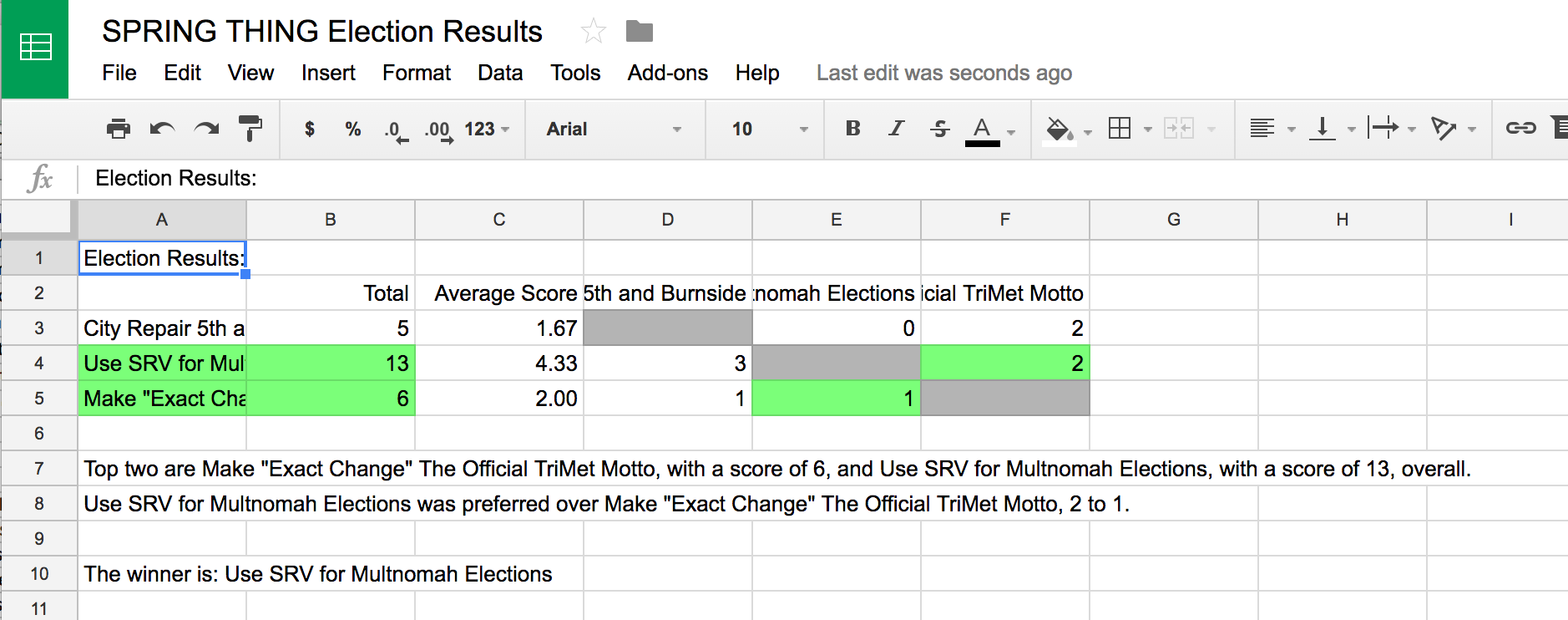# STAR Voting Election Calculator

## STAR Voting Election Calculator

### Due to recent changes in the Google API, the script described on this page is currently raising an error. Our software team is working on resolving the problem asap. We apologize for any inconvenience. In the meantime, if you prefer to use Google Forms to host your election you can still do so, you'll just need to tally the votes by hand.

STAR Voting now has it's own web app for running simple and functional STAR votes. Give it a try on star.vote

With Google Forms it's also fairly easy to set up and run your own STAR Voting* elections with additional security features, by copying and pasting in a simple script.

This post is a step-by-step guide that shows how to do that.

* Some images in this post say SRV, which reflects the original working name for STAR Voting. SRV stands for Score Runoff Voting. STAR Voting stands for Score Then Automatic Runoff.

Go to Google Forms and create a new form.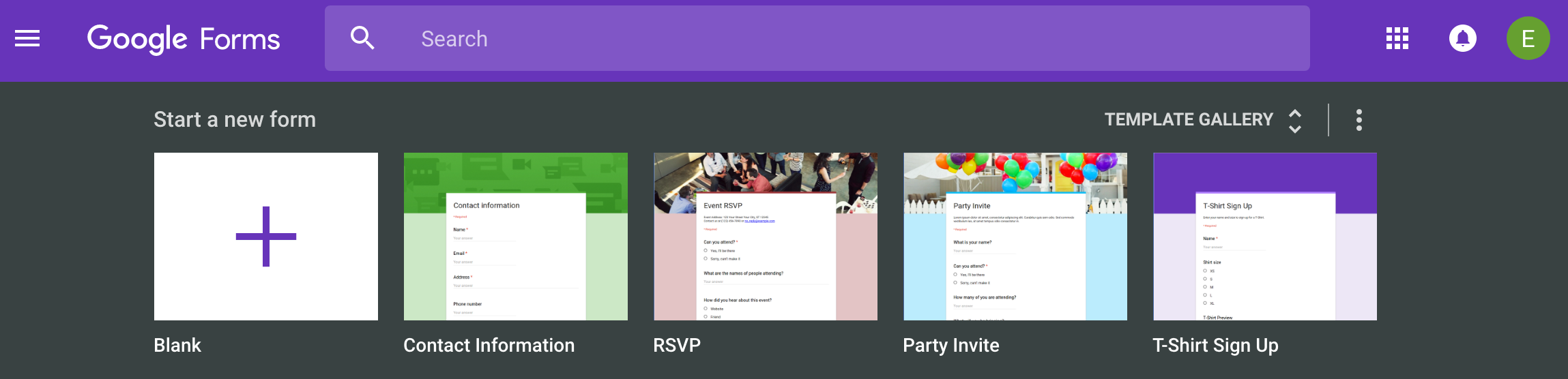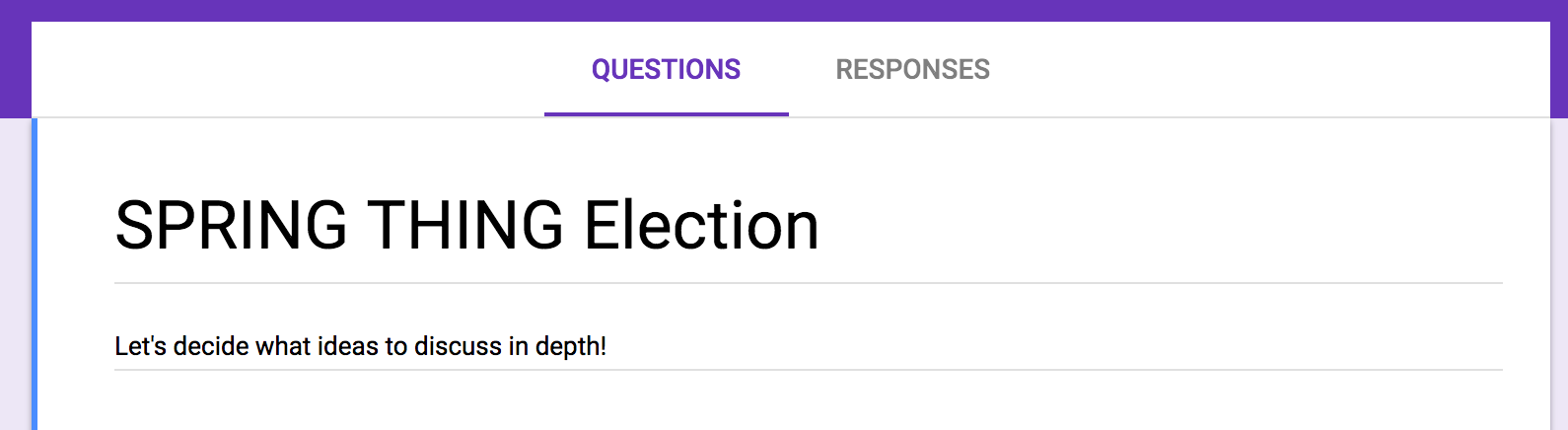Step 3: Choose the "Linear Scale" item type for the first entry

Each entry on the ballot should use the "Linear Scale" item type. This a 0-5 star style ballot.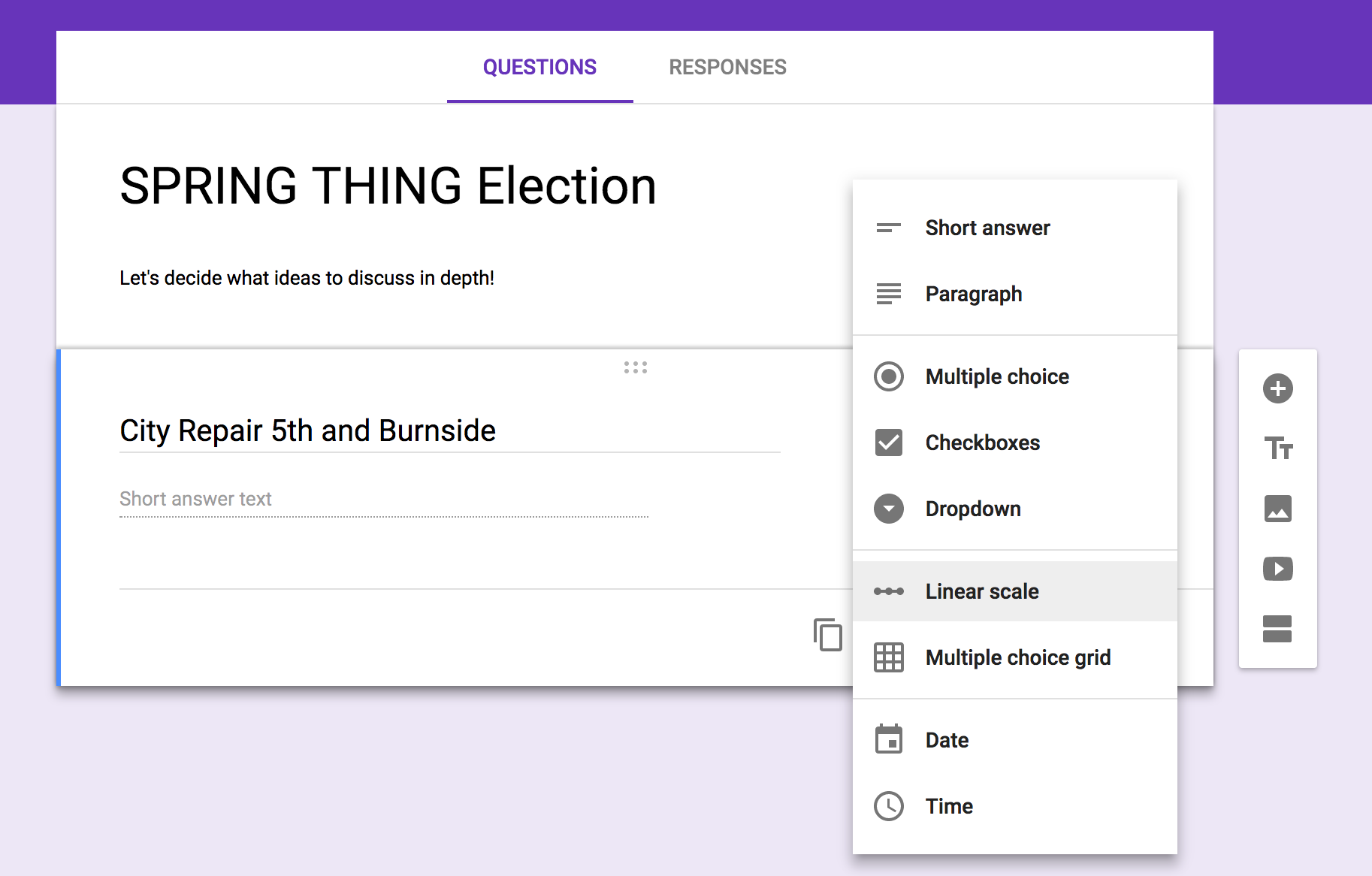Step 4: Set the scale start to 0

Set the scale to 0-5 on each ballot entry. Since Google Forms defaults to 1 as the starting value, you'll need to set it manually to 0.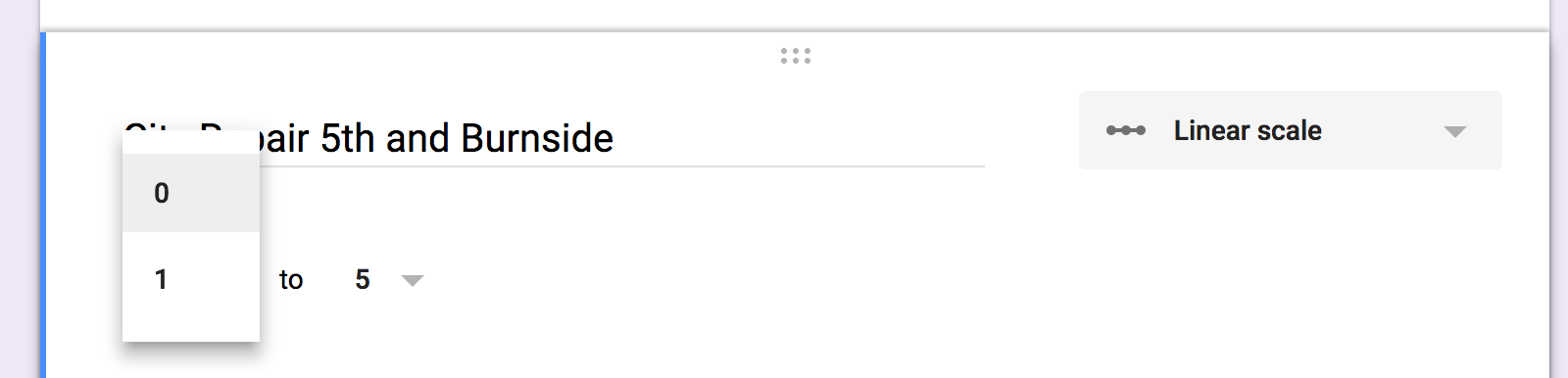Step 5: Make some more candidates on the ballot!

Once you have created the first candidate with the 0-5 linear scale you can copy it and then rename the next candidate.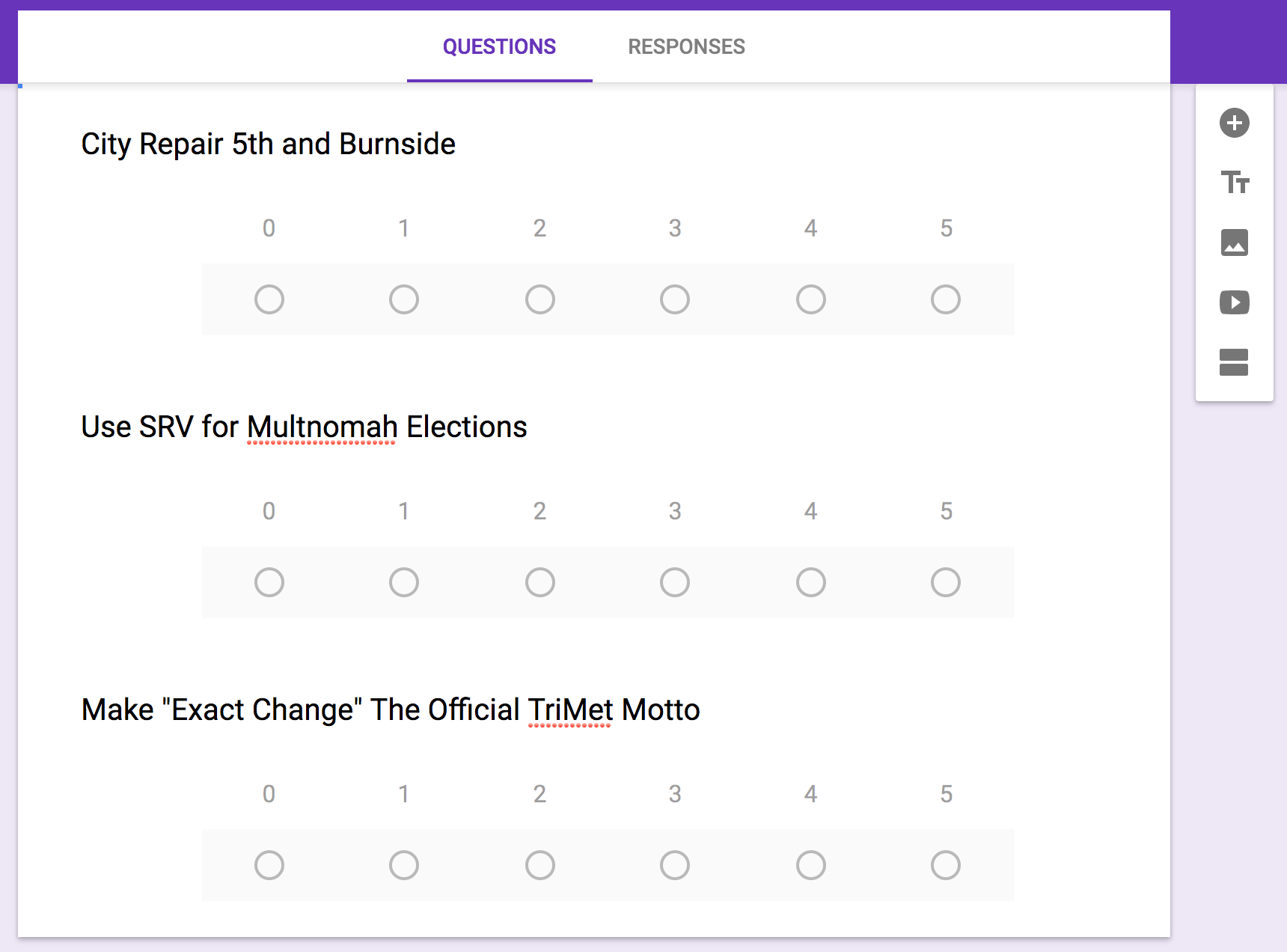Step 6: Open the Script Editor

From the action menu (the vertical "..." in the upper left), select the "< > Script Editor..." option. This is where you'll put the custom code to officiate the election from the form.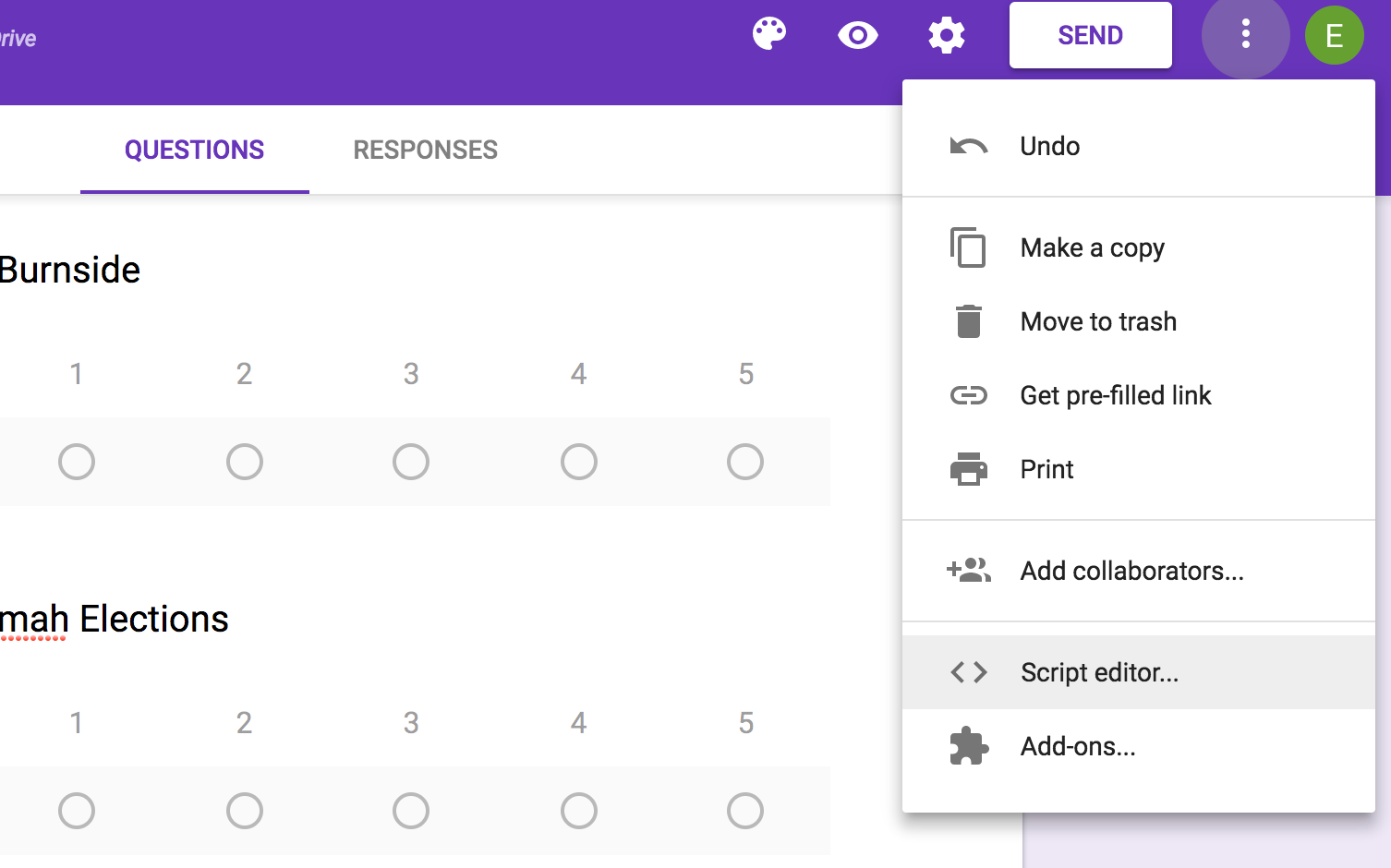Step 7: Replace all the code with the SRV calculator script

Copy the following code and paste it over the contents of the form's script.

``````
/** * @OnlyCurrentDoc */var ADDON_TITLE = 'STAR Voting Calculator';

function onOpen(e) {
FormApp.getUi()
}

function onInstall(e) {
onOpen(e);
}

function runElection() {
var form = FormApp.getActiveForm();
var responses = form.getResponses();
if(responses.length == 0)
return;

candidate_names = [];
formItems = form.getItems();
for (var i = 0; i < formItems.length; i++)
candidate_names[i] = formItems[i].getTitle();

num_candidates = formItems.length;

result_sheet.setName("Results");

result_range = result_sheet.getDataRange();
result_values = result_range.getValues();

num_ballots = responses.length;

// create empty score list and preference matrix
candidate_scores = [];
pref_matrix = [];
for(i = 0; i < num_candidates; i++)
{
candidate_scores[i] = 0;
pref_matrix[i] = [];
for(j = 0; j < num_candidates; j++)
pref_matrix[i][j] = 0;
}

for(b = 0; b < num_ballots; b++)
{
var ballot = responses[b].getItemResponses();
scores = [];
for(z = 0; z < num_candidates; z++)
scores[z] = 0;

for(i = 0; i < ballot.length; i++)
scores[ballot[i].getItem().getIndex()] = parseInt(ballot[i].getResponse());

for(c = 0; c < num_candidates; c++)
{
candidate_scores[c] += scores[c];
for(d = 0; d < num_candidates; d++)
if(scores[c] > scores[d])
pref_matrix[c][d] += 1;
}
}
function versus(x) { return 'vs. ' + x }
result_sheet.appendRow([ 'Election Results:' ]);
result_sheet.appendRow([ '', 'Total', 'Average Score'].concat(candidate_names.map( versus ) ) );
result_sheet.getRange(2, 2, 1, 2 + num_candidates).setHorizontalAlignment("right");

for(c = 0; c < num_candidates; c++)
{
pref_matrix[c][c] = '';
result_sheet.getRange(3 + c, 3).setNumberFormat("0.00");
result_sheet.getRange(3 + c, 4 + c).setBackgroundRGB(180, 180, 180);
}

// find the top two
top_a = 0;
top_b = 1;

for(c = top_b + 1; c < num_candidates; c++)
{
if(candidate_scores[c] > candidate_scores[top_a])
{
if(candidate_scores[top_a] > candidate_scores[top_b])
top_b = top_a;
top_a = c;
}
else if(candidate_scores[c] > candidate_scores[top_b])
{
top_b = c;
}
}
result_sheet.getRange(3 + top_a, 1, 1, 2).setBackgroundRGB(128, 255, 128);
result_sheet.getRange(3 + top_b, 1, 1, 2).setBackgroundRGB(128, 255, 128);
result_sheet.getRange(3 + top_a, 4 + top_b).setBackgroundRGB(128, 255, 128);
result_sheet.getRange(3 + top_b, 4 + top_a).setBackgroundRGB(128, 255, 128);

result_sheet.appendRow([' ']);
result_sheet.appendRow(['Top two are ' + candidate_names[top_a] + ", with a score of " + candidate_scores[top_a] + ", and " + candidate_names[top_b] + ", with a score of " + candidate_scores[top_b] + ", overall."]);
if(pref_matrix[top_a][top_b] >= pref_matrix[top_b][top_a])
{
winner = top_a;
loser = top_b;
}
else
{
winner = top_b;
loser = top_a;
}
result_sheet.appendRow([candidate_names[winner] + " was preferred over " + candidate_names[loser] + ", " + pref_matrix[winner][loser] + " to " + pref_matrix[loser][winner]+ "."] );
result_sheet.appendRow([' ']);
result_sheet.appendRow(['The winner is: ' + candidate_names[winner]]);
}
``````

When you're done it'll look like this: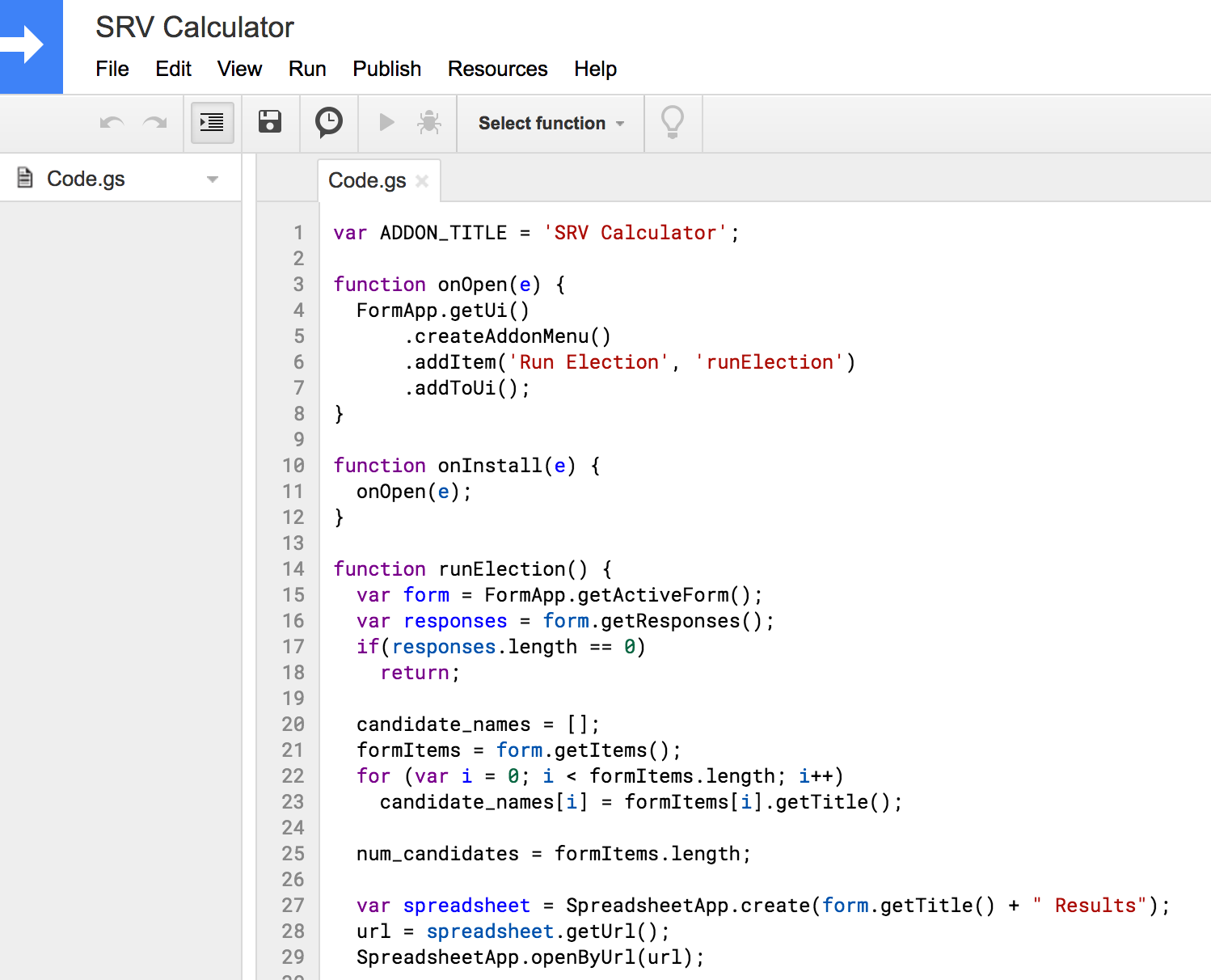Choose "Save" from the File menu. The script editor will ask you to save the script with a name. Call it "SRV Calculator:"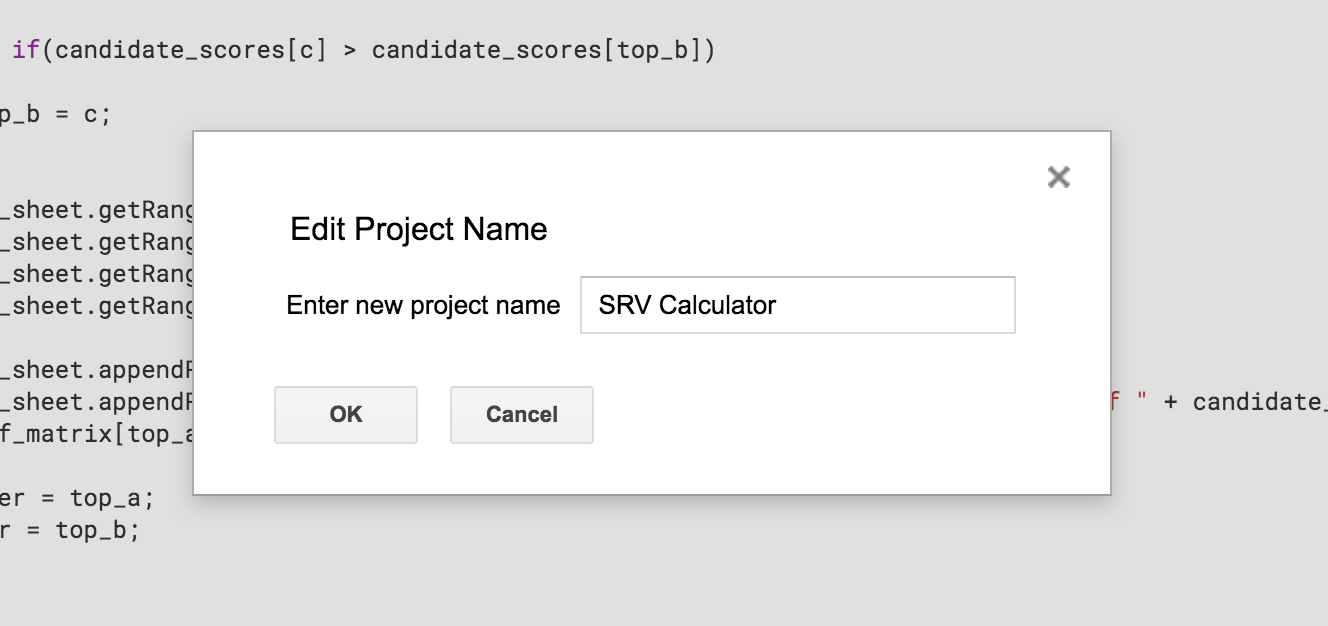Step 8: Send out the ballots!

You can send the ballots out in email form, or distribute a link to the ballot form via other means.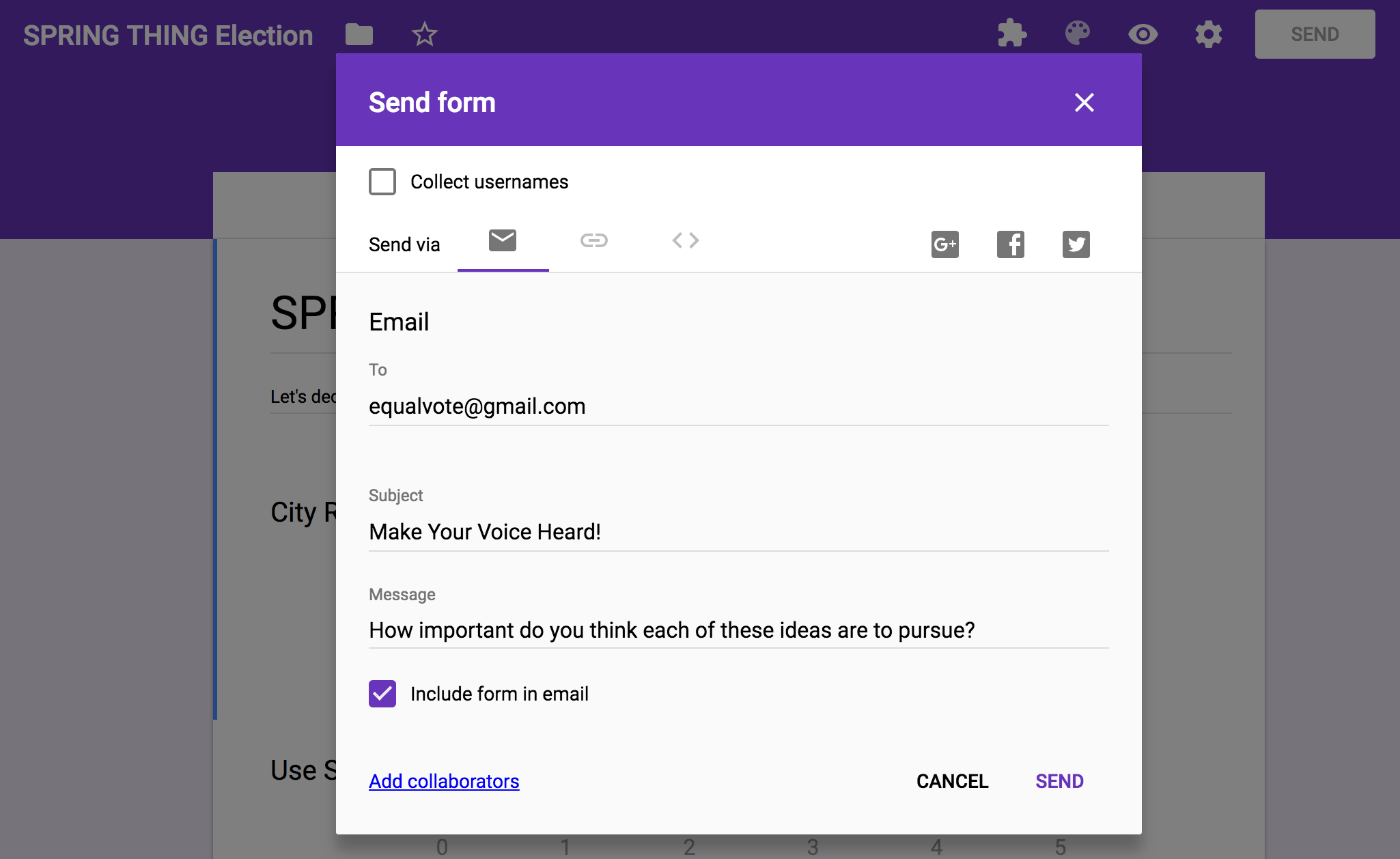If you checked the "Include form in email" option, users will be able to fill out the form within their email clients.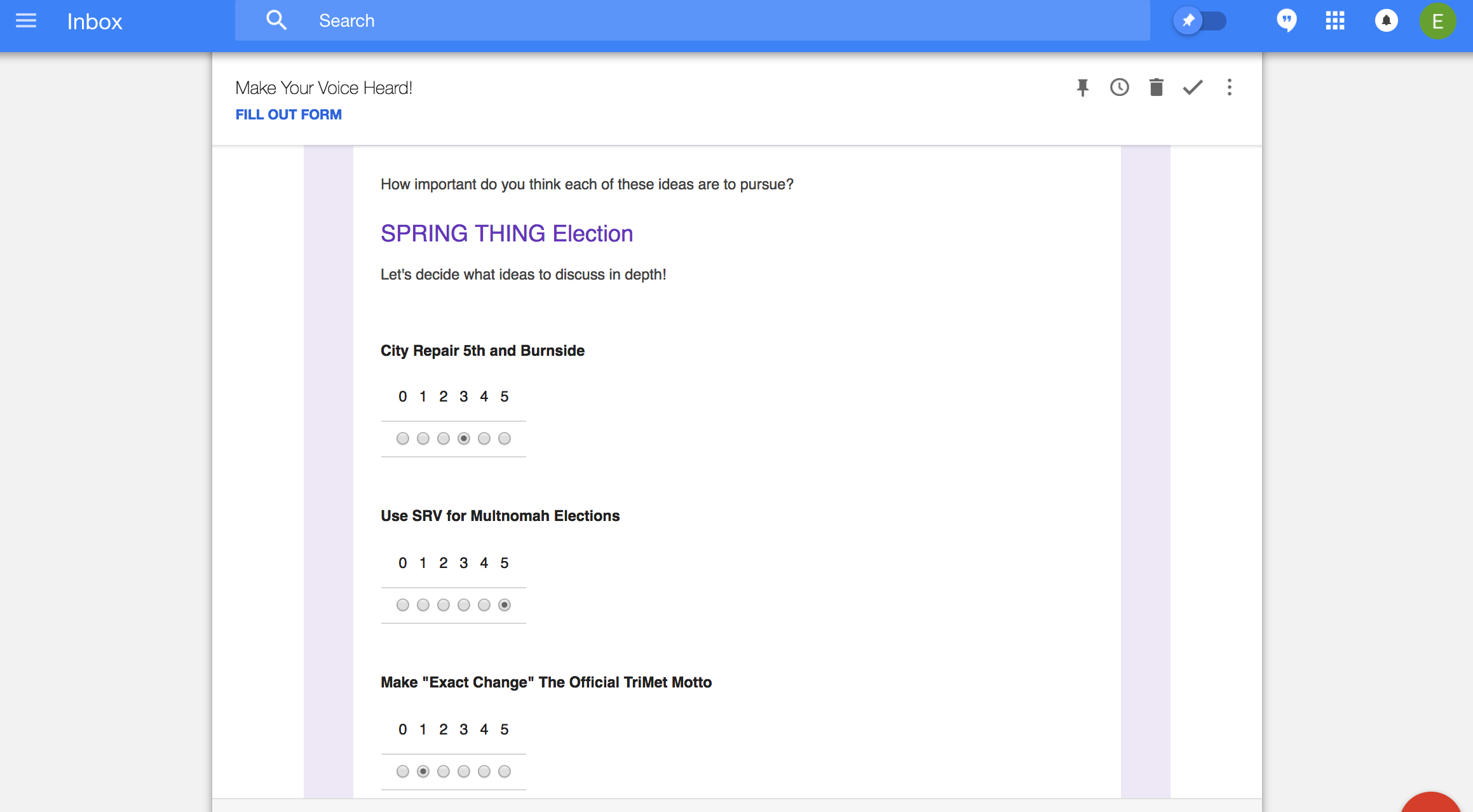Users can also fill out the form on the Google Forms site: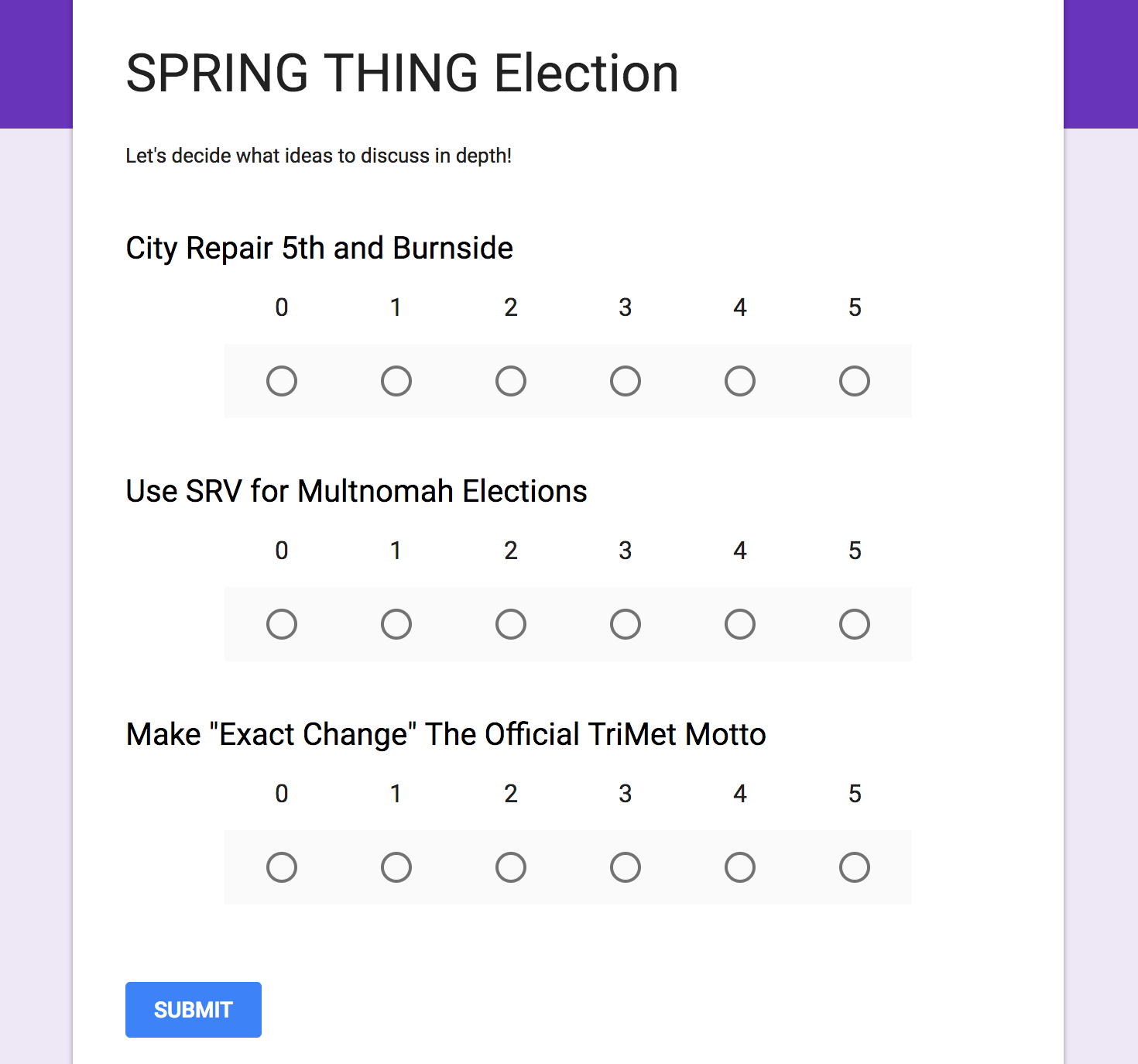Step 9: Run the election!

You'll need to close and re-open the form for the script option to show up in the Google Form. You can find the form easily in the "Recent" files section of your google drive: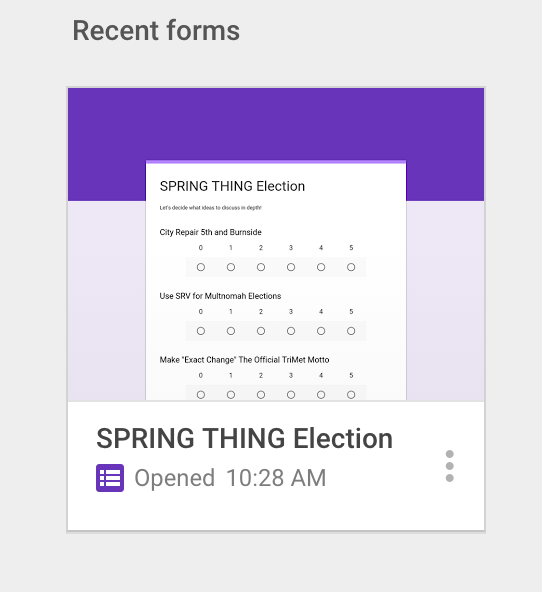Once you've re-opened the form, click the puzzle-piece add-ons icon and choose "SRV Calculator," and then choose "Run Election":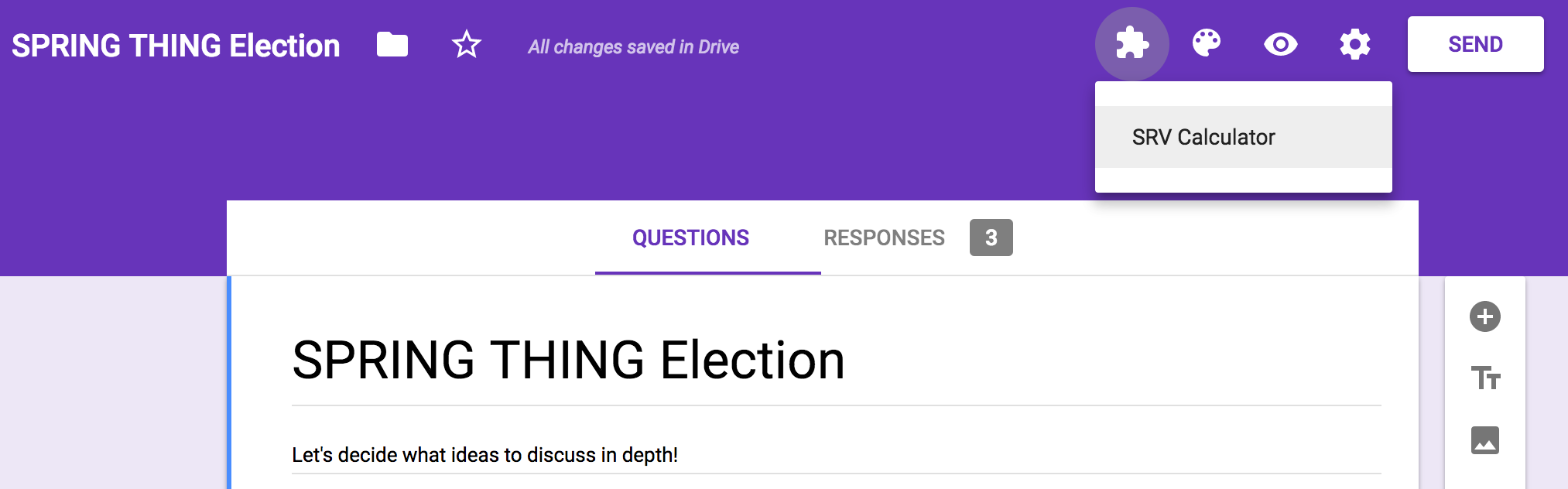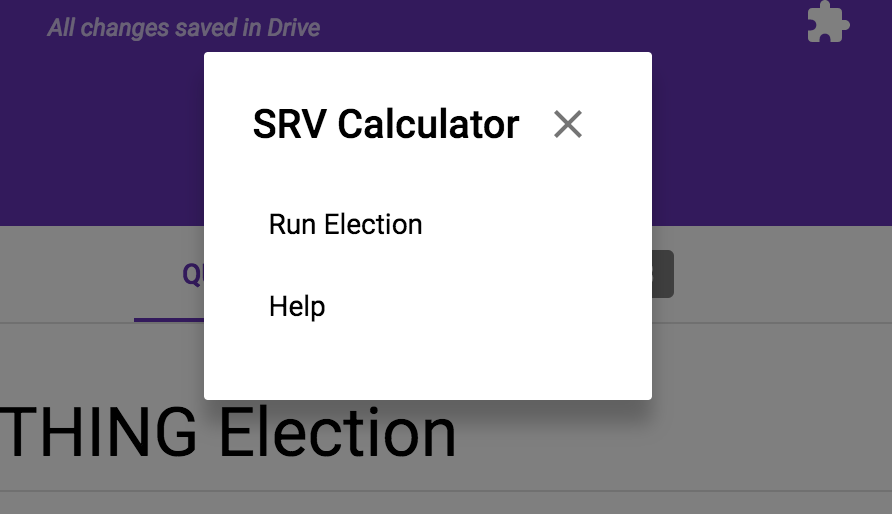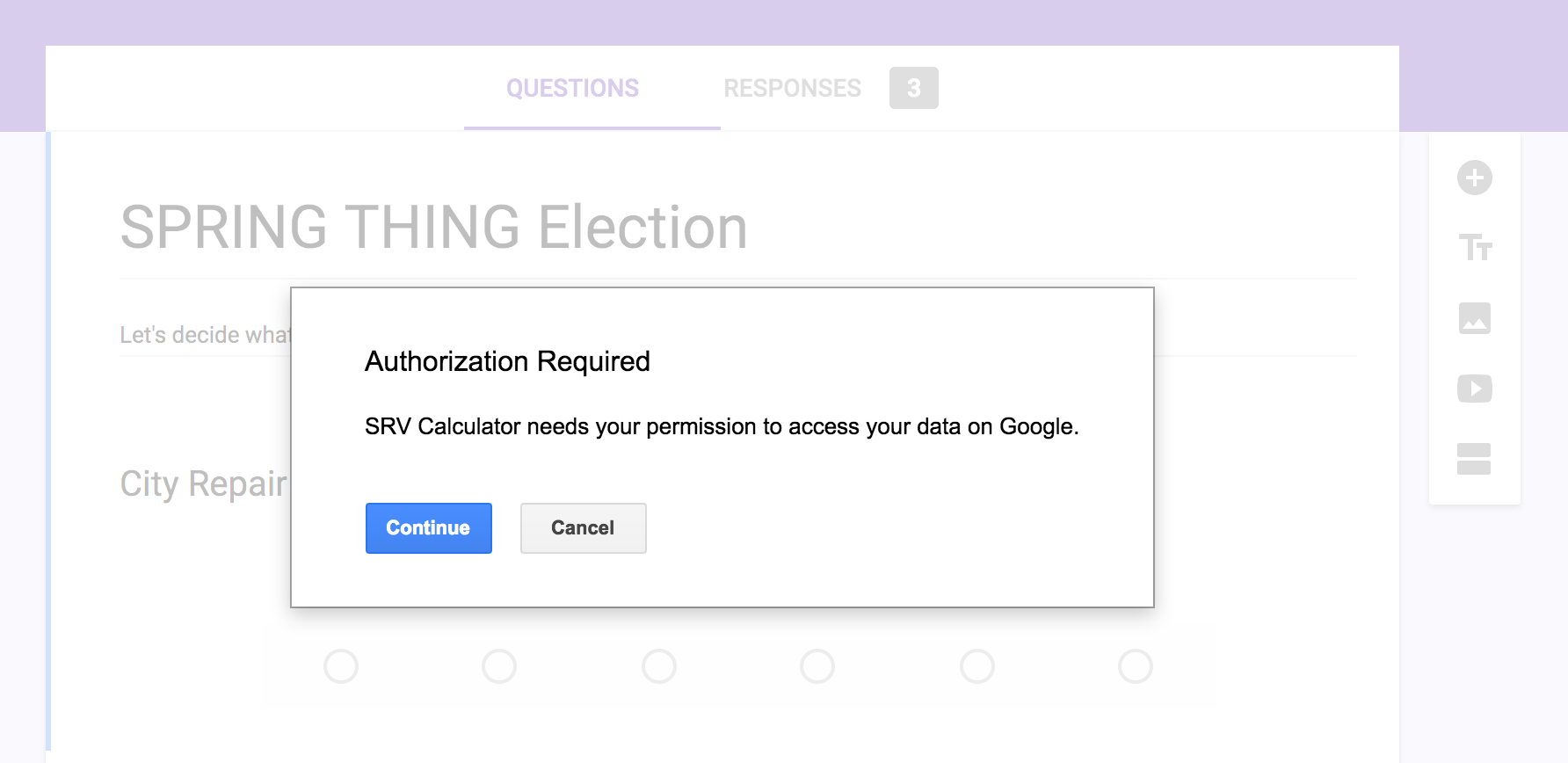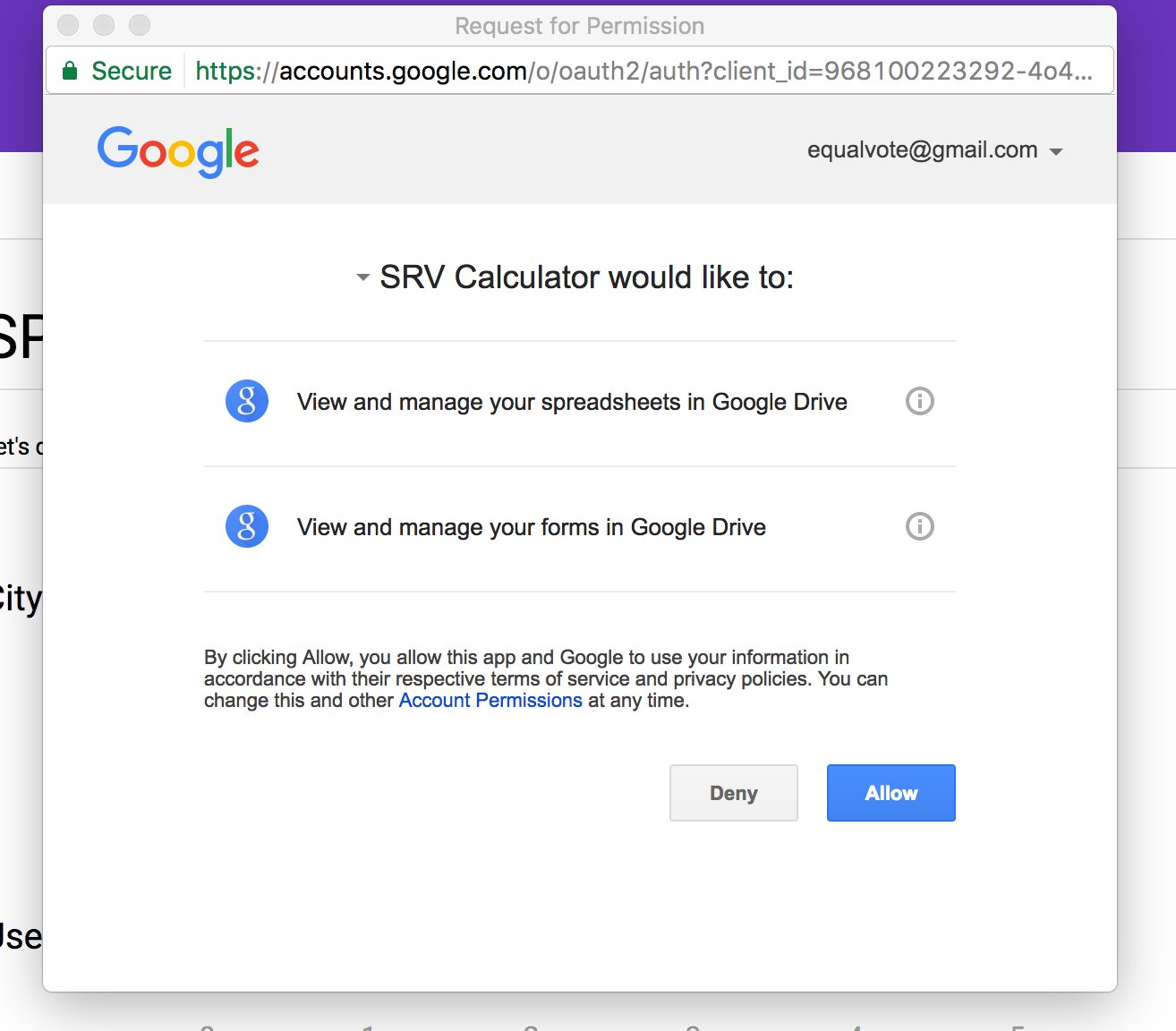Once the script has permission to run, a small dialog at the top of the page will show the progress. Once it's finished, the results page will be complete.

Step 10: Open the results

The SRV Calculator script creates a Google Sheet spreadsheet with the results of the election. You can find it most easily in the "Recent" files section in your Google Drive: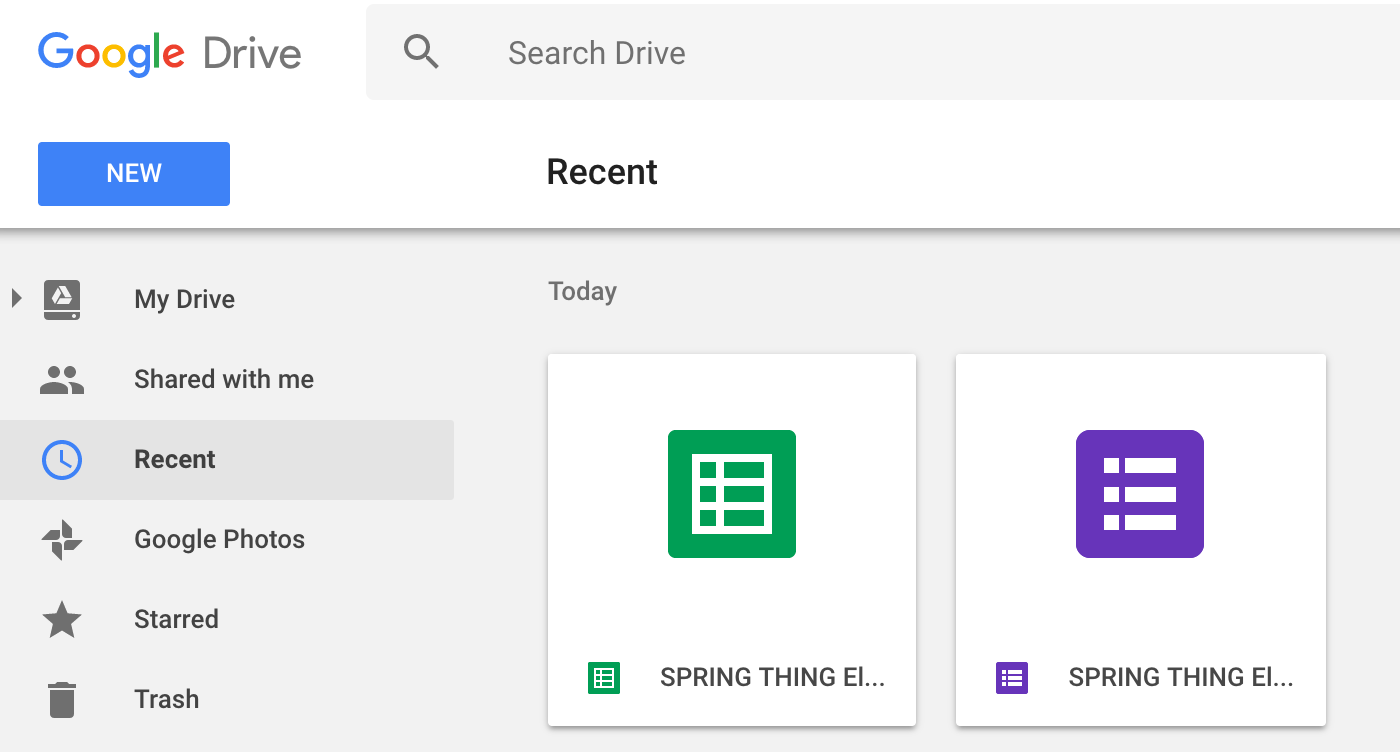Open the spreadsheet and see the results!